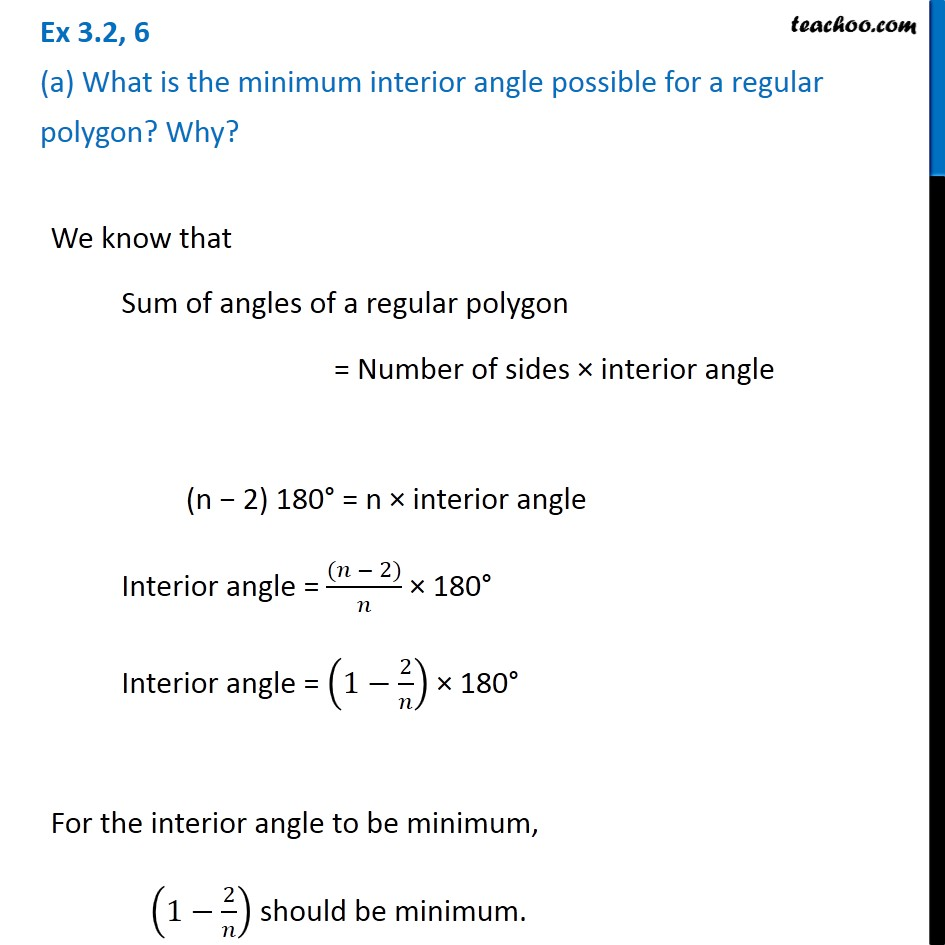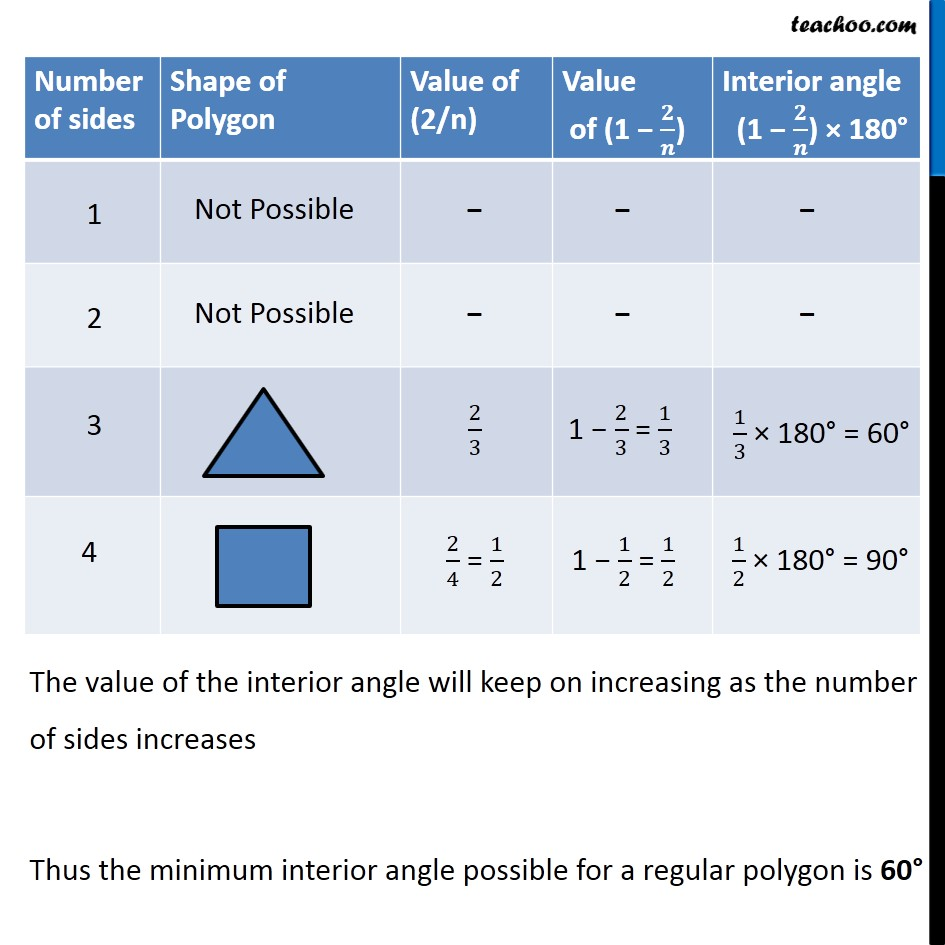Sum of Exterior Angles of PolygonsLearn in your speed, with individual attention - Teachoo Maths 1-on-1 Class

### Transcript

Ex 3.2, 6 (a) What is the minimum interior angle possible for a regular polygon? Why?We know that Sum of angles of a regular polygon = Number of sides × interior angle (n − 2) 180° = n × interior angle Interior angle = ((𝑛 − 2))/𝑛 × 180° Interior angle = (1−2/𝑛) × 180° For the interior angle to be minimum, (1−2/𝑛) should be minimum. The value of the interior angle will keep on increasing as the number of sides increases Thus the minimum interior angle possible for a regular polygon is 60°